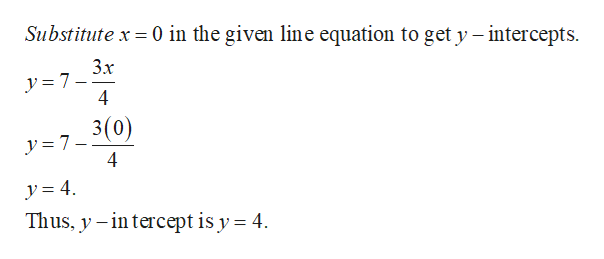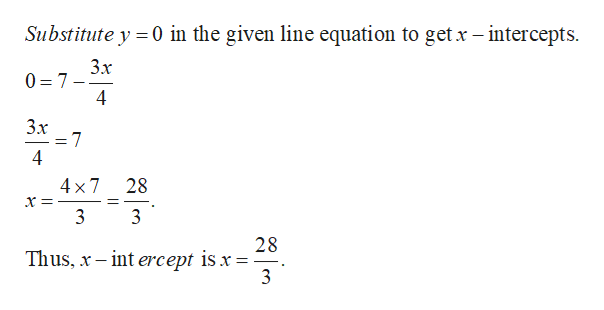Question
1 views

How do I graph y=7-3x/4?

check_circle

Step 1

Note that given equation represents line.help_outlineImage TranscriptioncloseSubstitute x = 0 in the given line equation to get y – intercepts. Зх y = 7- 4 3(0) y = 7– 4 y = 4. Thus, y – in tercept is y = 4. fullscreen
Step 2help_outlineImage TranscriptioncloseSubstitute y 0 in the given line equation to get x- intercepts 3x 0 7 4 3x 4 x 7 28 = . 28 Thus, x-int ercept is x = 3 4 fullscreen

### Want to see the full answer?

See Solution

#### Want to see this answer and more?

Solutions are written by subject experts who are available 24/7. Questions are typically answered within 1 hour.*

See Solution
*Response times may vary by subject and question.
Tagged in

### Math